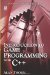# 8.2 Pointers

## 8.2 Pointers

Memory addresses are unique integers, so 845840 might be the memory address of a Boolean variable, and 9575786 could be the address of an integer variable. Pointers are variables that store the memory addresses of other variables in this way. So, if an integer variable called I is declared, a pointer called P can be declared to hold the memory address of I. As mentioned, the possibilities this has and the significance of knowing this might not immediately strike the reader as useful; after all, who cares whether the memory address of a variable is known as long as it works and values can be written or read as required? To answer this completely requires an examination of subjects that are discussed in later chapters. So for now, it is enough to know that pointers can hold the memory address of other variables. Pointers are declared using the indirection operator (*) and have the following form.

`          DataType *Number; `

Or:

`          DataType *Number = Address; `

### 8.2.1 Declaring Pointers

Pointers store the memory address of other variables. They are declared much like any other variable, except that the indirection operator (*) is used to distinguish pointers from ordinary variables. Consider the following code:

`      int *Pointer_To_Int;      int number_variable = 0;      char *this_is_a_pointer_to_a_char; `
 Note Only two pointers are declared in this sample: int *Pointer_To_Int and char *this_is_a_pointer_to_a_char. One is set to point to an integer variable, and the other is set to point to a char variable. Like variables, pointers are initialized to default starting values, and so these pointers currently point nowhere, since no memory address has been assigned to them yet.

It is understandable to think the memory address of a variable can be assigned to a pointer in this way:

`      int variable = 0;      int *pointer = variable; `

However, this is not correct. Doing this will assign the value of the variable to the pointer. Remember, the value of the variable is the actual data being held at a specific location in memory. The intention, then, is not to assign the actual value of the variable to the pointer, but the numerical address in memory where the value is being held. To take the address of a variable, the Address Of & (ampersand) operator is used. The above code then can be written correctly as follows:

`      int variable = 0;      int *pointer = &variable; `

### 8.2.3 Pointer Dereferencing

Given a pointer, is it possible to obtain the value held at that memory address? The answer is yes, and this process is called pointer dereferencing. To do this, the indirection operator must again be used. Consider the following sample code:

`      int variable = 5;      int *pointer = &variable;      int variable2 = *pointer; //value at address is assigned `

### 8.2.4 Pointer Arithmetic

Since pointers represent numerical memory addresses, they are subject to the rules of arithmetic. To add, subtract, multiply, or divide a number and a pointer means to change the memory address it represents. Consider the following sample code:

`      int variable = 0;      int *pointer= &variable;      int *pointer2 = pointer+15; //references new location `
 Note Use pointer arithmetic with caution, as it can lead to unexpected results. If a location is referenced that is not valid, a segment fault error is raised; in other words, it's likely your application will crash.

### 8.2.5 Pointers and Arrays

Arrays, as mentioned, are linear lists of elements in memory. To access an element, the array subscript operator ([]) can be used. For example, an array of integers can be declared and accessed as follows:

`      int Numbers[] = [5, 10, 15, 20, 25, 30, 35, 40};      int Number1 = Numbers; //first element      int Number2 = Numbers; //second element      int Number3 = Numbers; //third element `

However, pointers can also be used to access array elements using pointer arithmetic. This can easily be proved since an array is a linear list of items in memory; in other words, the first item in an array will be arranged in memory to appear before the next item, and so on. The next section examines how to do this.

### 8.2.6 Using Pointers and Arrays

Consider the following array: int Numbers. Using the subscript operator, the first element is accessed as Numbers. However, when Numbers appears alone, without the subscript operator, it can be seen as a pointer to the first element in the array. So, int *FirstElement = Numbers. Consequently, the following code can be considered:

`      #include <iostream>      int main()      {         int Numbers[] = {1, 2, 3, 4, 5};         int *Pointer_To_Number = Numbers;         std::cout<<*Pointer_To_Number; //First number         Pointer_To_Number++; //Add 1 to pointer for next number         std::cout<<*Pointer_To_Number; //Second number         Pointer_To_Number++; //Add 1 to pointer for next number         //And so on         return 0;      } `Introduction to Game Programming with C++ (Wordware Game Developers Library)
ISBN: 1598220322
EAN: 2147483647
Year: 2007
Pages: 225
Authors: Alan Thorn

Similar book on Amazon